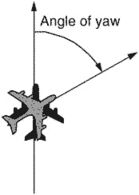# yaw angle

Also found in: Dictionary, Wikipedia.

## yaw angle

[′yȯ ‚aŋ·gəl]
(aerospace engineering)
McGraw-Hill Dictionary of Scientific & Technical Terms, 6E, Copyright © 2003 by The McGraw-Hill Companies, Inc.

## angle of yawThe angle between a line in the direction of flight and a plane through the longitudinal and vertical axes of an aircraft. It is considered to be positive if the nose is displaced to the right and negative if displaced to the left. Also called a yaw angle.
Mentioned in ?
References in periodicals archive ?
Section 4 proposes different feature based driver distraction techniques containing different features for the detection of driver's head rotation (due to change in yaw angle) using different classifiers including Neural Network, Naive Bayes, SVM, Adaboost, and Decision Trees.
The bigger the wheel diameter difference, the greater the wheel/rail longitudinal creep force, so is the wheelset yaw angle. Thus, the wheel/rail lateral creep force caused for above reason is become greater, too.
Maximum body yaw angle 4.9[degrees] after application of the system has been registed for12 m full bus.
The equation rotates each laser detected data point about the w-axis at the offset yaw angle ([gamma]) .
Figure 16 depicts the simulation curve with body pitch angle and yaw angle input.
Figure 2 shows the expected and actual position and yaw angle curves.
The control objective for the quadrotor is to track a desired trajectory and a desired yaw angle. The composition of this method is as follows: First, a backstepping procedure is used to derive the stabilizing functions.
Although the flat-based 7.62x39mm bullet is shorter (0.930-inch) than the more common boattail projectile (1.040-inch), it will be expected to cause more damage to the abdomen, liver, spleen or pancreas because the bullet passes through these organs at a large yaw angle. Remember, if we have neither mushrooming nor fragmentation, yawing is all that remains to maximize tissue disruption and enhance the bullet's performance--always provided we do not sacrifice adequate penetration.
Due to 10dof sensor measurement noise, mechanical misalignments in mirror mounting, imperfections of 3D printing, a center of mass offset the definition of the pitch angle (relative to the yaw angle) was less accurate.
The rule is interpreted as follows: (1) nonacceleration mode: when [[alpha].sub.k] = 0, there is no vehicle acceleration, and the yaw angle can be determined by the acceleration and magnetometers; (2) low-acceleration model: when [[alpha].sub.k] = 1, the dynamic acceleration could be regarded as a part of measurement noise; (3) high-acceleration model: when [[alpha].sub.k] = [infinity], the accelerometers measurements are far from the value of the gravity, and then, the yaw angle calculated by (1b) is far away from the truth and the yaw angle is actually determined by the gyros .

Site: Follow: Share:
Open / Close